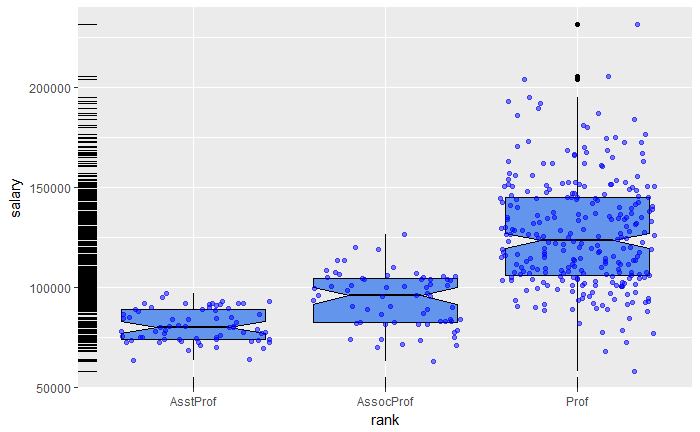# 1. 四种图形系统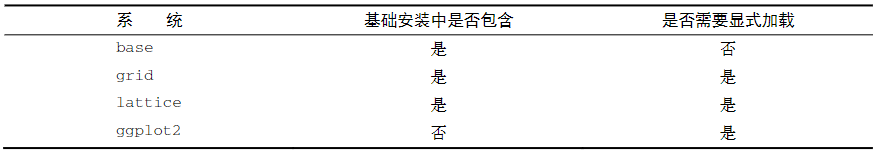• 第一个是从lattice包中的singer数据集，它包括纽约合唱团歌手的高度和语音变量。
• 第二个是在本书中已经使用过的mtcars数据集，它包含32辆汽车的详细信息。
• 最后一个是在第8章中讨论的car包中的Salaries数据集。Salaries数据集包含大学教授的收入信息，并用来探索性别差异对他们收入的影响。

# 2. ggplot2 包介绍

• 示例1：简单示例
1. ggplot初始化图形并指定要用到的数据来源data,和变量aes(aes:aesthetics 视觉呈现信息)
2. aes()函数指定每个变量扮演的角色
3. geom_point()表示创建一个散点图
4. labs()函数是可选函数，可添加注释(包括轴标签和标题)
``````ggplot(data=mtcars,aes(x=wt,y=mpg)) +
geom_point() +
labs(title="Automobile Data",x="Weight",y="Miles Per Gallon")
``````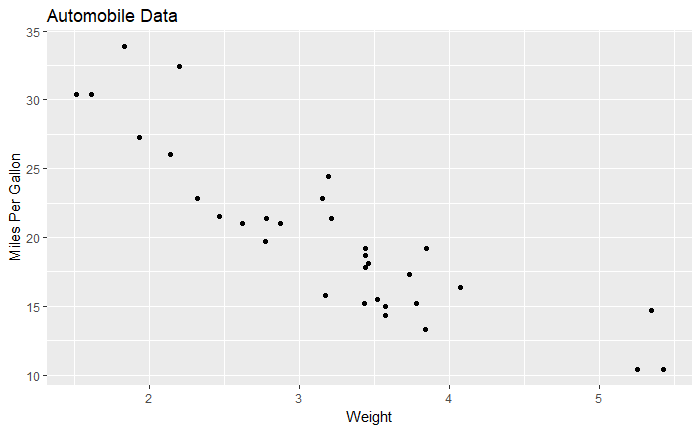• 示例2
1. geom_point函数中，pch=17表示三角形，size=2点的大小加倍
2. geom_smooth()函数增加了一条平滑的曲线，这里需要线性拟合(method=”lm”),红色的虚线
``````ggplot(data=mtcars,aes(x=wt,y=mpg)) +
geom_point(pch=17,color="blue",size=2) +
geom_smooth(method="lm",color="red",linetype=2) +
labs(title="Automobile Data",x="Weight",y="Miles Per Gallon")
``````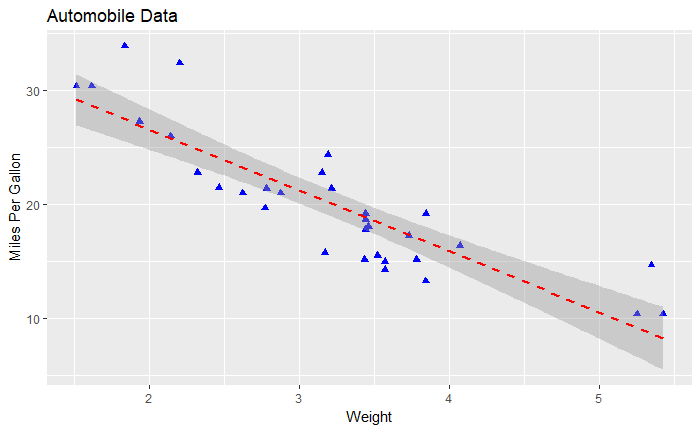• 示例3：分组和小面化(faceting)

``````mtcars\$am <- factor(mtcars\$am,levels = c(0,1),
labels = c("Automatic","Manual"))

mtcars\$vs <- factor(mtcars\$vs,levels = c(0,1),
labels = c("V-Engine","Straght Engine"))

mtcars\$cyl <- factor(mtcars\$cyl)
``````
``````ggplot(data=mtcars,aes(x=hp,y=mpg,shape=cyl,color=cyl)) +
geom_point(size=3) +
facet_grid(am~vs) +
labs(title="Automobile Data by Engine Type",x="Horsepower",y="Miles Per Gallon")

``````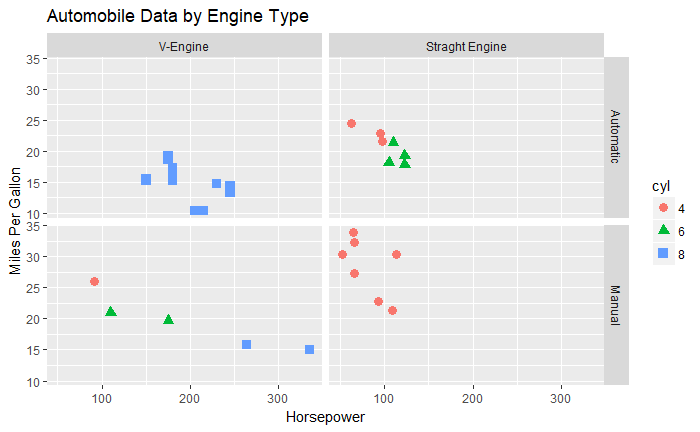# 3. 用几何函数指定图的类型

ggplot()函数指定要绘制的数据源和变量，几何函数则指定这些变量如何在视觉上进行表示(使用点/条/线/阴影区)，下面是常见的几何函数。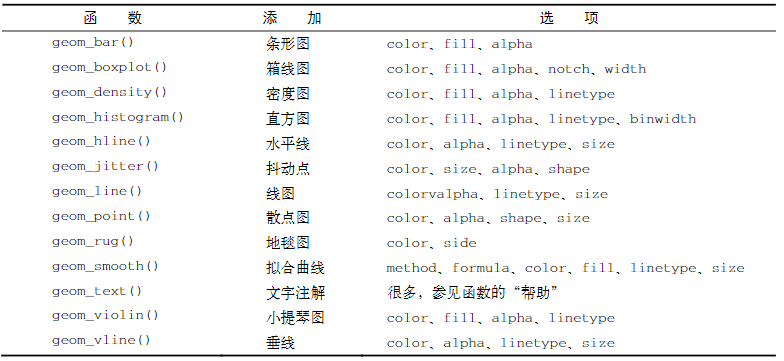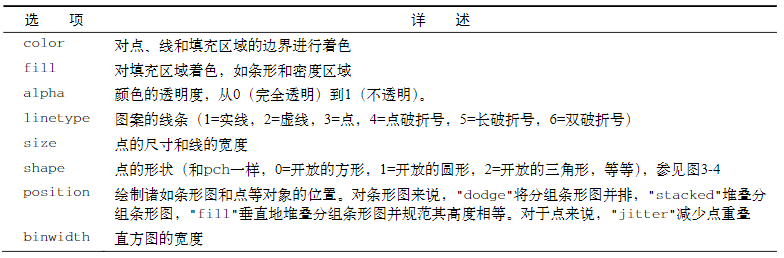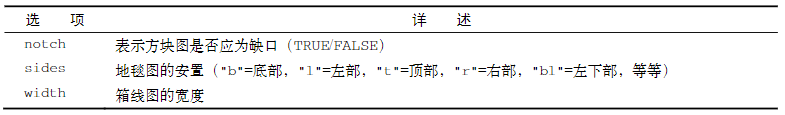• 示例1：
``````data(singer,package = "lattice")

ggplot(data=singer,aes(x=height)) + geom_histogram()
``````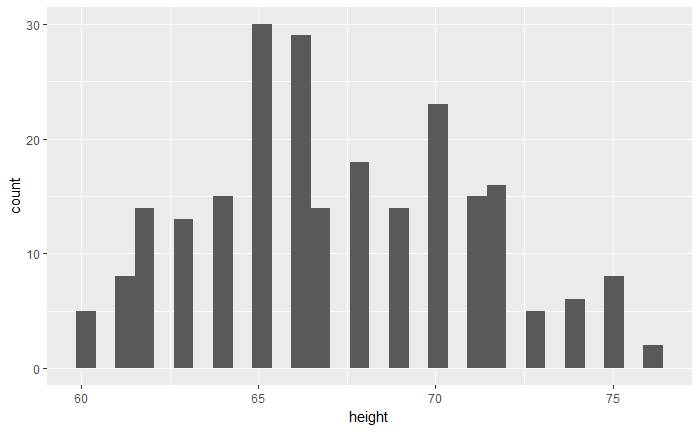• 示例2：
``````ggplot(data=singer,aes(x=voice.part,y=height)) + geom_boxplot()
``````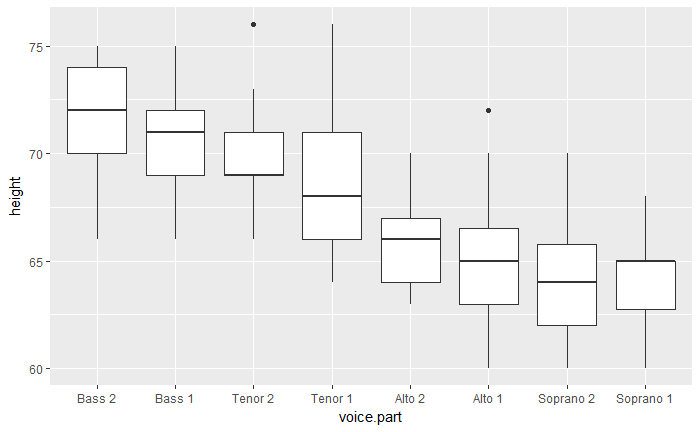• 示例3，不同学术地位对应薪水的缺口箱线图。
1. 实际的观测值(教师)是重叠的，因而给了一定的透明度0.5.
2. 使用了抖动jitter以减少重叠。
3. 最后一个地毯图在左侧显示薪水的一般扩散。
``````#install.packages("car")
data(Salaries,package = "car")
``````
``````ggplot(data=Salaries,aes(x=rank,y=salary)) +
geom_boxplot(fill="cornflowerblue",
color="black",notch=TRUE) +
geom_point(position = "jitter",color="blue",alpha=.5) +
geom_rug(side="1",color="black")
``````